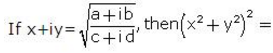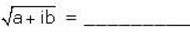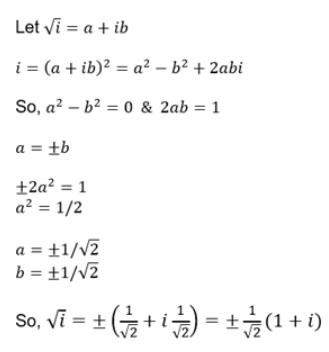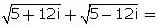Courses

# Test: Square Root Of A Complex Number

## 5 Questions MCQ Test Mathematics For JEE | Test: Square Root Of A Complex Number

Description
This mock test of Test: Square Root Of A Complex Number for JEE helps you for every JEE entrance exam. This contains 5 Multiple Choice Questions for JEE Test: Square Root Of A Complex Number (mcq) to study with solutions a complete question bank. The solved questions answers in this Test: Square Root Of A Complex Number quiz give you a good mix of easy questions and tough questions. JEE students definitely take this Test: Square Root Of A Complex Number exercise for a better result in the exam. You can find other Test: Square Root Of A Complex Number extra questions, long questions & short questions for JEE on EduRev as well by searching above.
QUESTION: 1

###Solution:

(x+iy)(x−iy) = (a+ib)(a−ib)/(c+id)(c−id)
⇒x2−iy2 = √[(a2−i2b2)/(c2−i2d2)]
⇒x2+y2 = √[(a2+b2)/(c2+d2)]  [1i2 = -1]
(x2+y2)2 = (a2+b2)/(c2+d2)

QUESTION: 2

###Solution:

(a + ib)1/2 = (x + iy)
Squaring both sides,
a + ib = (x + iy)2
a + ib = x2 - y2 + 2ixy
Equating real and imaginary
a = x2 - y2    b = 2xy............(1)
Using (x2 + y2)2 = (x2 - y2)2 + 4xy
(x2 + y2)2 = a2 + b2
(x2 + y2) = (a2 + b2)1/2.......(2)
2x2 = (a2 + b2)1/2 + a
x = +-{1/2(a2 + b2)1/2 + a}1/2
Subtract (2) from (1)
2y2 = (a2 + b2)1/2 - a
y = x = +-{1/2(a2 + b2)1/2 - a}1/2
Therefore, (a+ib)1/2 = x+iy
=> +-{1/2(a2 + b2)1/2 + a}1/2 + i+-{1/2(a2 + b2)1/2 - a}1/2

QUESTION: 3

### Square root of 5 + 12i is

Solution:

(a + ib)2 = 5 + 12i
use (a + b)2 = a2 + b2 + 2*a*b
=> a2 + b2*i2 + 2*a*b*i = 5 + 12i
i2 = -1
⇒ a2 - b2 + 2*a*b*i = 5 + 12i
equate the real and complex coefficients
⇒ a2 - b2 = 5 and ab = 6
a = 6/b
substitute in a2 - b2 = 5
⇒ 36/b2 - b2 = 5
⇒ 36 - b4 = 5b2
⇒ b4 + 5b2 - 36 = 0
⇒ b4 + 9b2 - 4b2 - 36 = 0
⇒ b2(b2 + 9) - 4(b2 + 9) = 0
⇒ (b2 - 4)(b2 + 9) = 0
⇒ b2 = 4 and b2 = -9
b is a real number, so we eliminate b2 = -9
b2 = 4
⇒ b = 2 and b = -2
a = 3 and a = -3
The required number can be 3 + 2i and -3 -2i

QUESTION: 4

The square root of i is

Solution:QUESTION: 5Solution:

Let √(5 – 12i) = x + iy
Squaring both sides, we get
5 – 12i = x2 + 2ixy +(iy)2 = x2 – y2 + 2xyi.
Comparing real and imaginary parts , we get
5 = x2 – y2 ———– (1) and xy = – 6 ———— (2)
Squaring (1), we get
25 = (x2 – y2)2 = (x2 + y2)2 – 4x2y2
⇒ 25 = (x2 + y2)2 – 4(– 6)2
⇒ (x2 + y2)2 = 169
⇒ x2 + y2 = 13 ———- (3)
Adding (1) and (3) we get
2x2 = 18
⇒ x = ± 3.
Subtracting (1) from (3) we get
2y2 = 8
⇒ y = ± 2.
Hence, square root of √(5 – 12i) is (3 – 2i)
Similarly, √(5 + 12i) is (3 + 2i)
√(5 + 12i) + √(5 – 12i)
⇒ (3 + 2i) + (3 - 2i)
⇒ 6